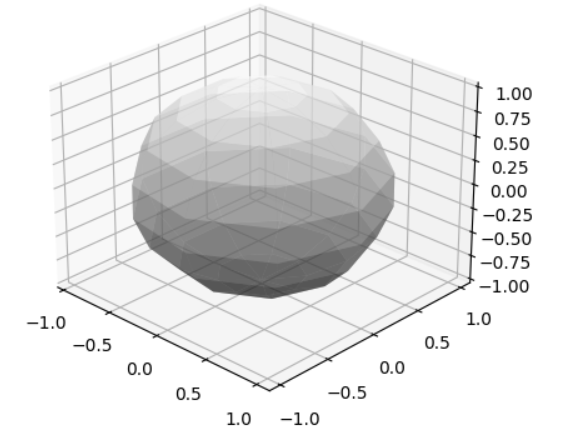• Python画球面
2020-11-20 22:35:50

如何在Python中绘制一个球面段，特别是一个球面“片”？from mpl_toolkits.mplot3d import Axes3D

import matplotlib.pyplot as plt

import numpy as np

fig = plt.figure()

ax = fig.add_subplot(111, projection='3d')

u = np.linspace(0, 2 * np.pi, 100)

v = np.linspace(0, np.pi, 100)

x = 10 * np.outer(np.cos(u), np.sin(v))

y = 10 * np.outer(np.sin(u), np.sin(v))

z = 10 * np.outer(np.ones(np.size(u)), np.cos(v))

ax.plot_surface(x, y, z, rstride=4, cstride=4, color='b')

plt.show()

如果我通过操作u和v的定义来改变上述代码，例如：u = np.linspace(0, 2 * np.pi, 20)

v = np.linspace(0, np.pi, 20)

球体仍然作为一个整体呈现，但分辨率很差。改变空间范围的起点似乎没有任何改变。

更多相关内容
• (具体地说，这些点是Lebedev求积点)我希望我的图看起来类似于我在网上找到的这个：我继续绘制一个球面，然后用散点图覆盖它。然而，这导致我的大部分观点被潜在的领域“吸收”，使它们很难被看到。看一看：我怎样...

我正在尝试生成一个球体的绘图，其中一些点绘制在球体的表面上。(具体地说，这些点是Lebedev求积点)我希望我的图看起来类似于我在网上找到的这个：我继续绘制一个球面，然后用散点图覆盖它。然而，这导致我的大部分观点被潜在的领域“吸收”，使它们很难被看到。看一看：我怎样才能防止我的点被球体遮住呢？以下是我用来生成此绘图的脚本：import matplotlib.pyplot as plt

from matplotlib import cm, colors

from mpl_toolkits.mplot3d import Axes3D

import numpy as np

# Create a sphere

r = 1

pi = np.pi

cos = np.cos

sin = np.sin

phi, theta = np.mgrid[0.0:pi:100j, 0.0:2.0*pi:100j]

x = r*sin(phi)*cos(theta)

y = r*sin(phi)*sin(theta)

z = r*cos(phi)

#Import data

data = np.genfromtxt('leb.txt')

xx, yy, zz = np.hsplit(data, 3)

#Set colours and render

fig = plt.figure()

ax = fig.add_subplot(111, projection='3d')

ax.plot_surface(

x, y, z, rstride=1, cstride=1, color='c', alpha=0.6, linewidth=0)

ax.scatter(xx,yy,zz,color="k",s=20)

ax.set_xlim([-1,1])

ax.set_ylim([-1,1])

ax.set_zlim([-1,1])

ax.set_aspect("equal")

plt.tight_layout()

#plt.show()

编辑

我已经找到了一种使用Python的mayavi来实现这一点的方法。我得到的是：下面是我使用的代码：from mayavi import mlab

import numpy as np

# Create a sphere

r = 1.0

pi = np.pi

cos = np.cos

sin = np.sin

phi, theta = np.mgrid[0:pi:101j, 0:2 * pi:101j]

x = r*sin(phi)*cos(theta)

y = r*sin(phi)*sin(theta)

z = r*cos(phi)

mlab.figure(1, bgcolor=(1, 1, 1), fgcolor=(0, 0, 0), size=(400, 300))

mlab.clf()

data = np.genfromtxt('leb.txt')

xx, yy, zz = np.hsplit(data, 3)

mlab.mesh(x , y , z, color=(0.0,0.5,0.5))

mlab.points3d(xx, yy, zz, scale_factor=0.05)

mlab.show()

展开全文• ## python matplotlib画球

千次阅读 2021-09-17 14:37:04
matplotlib球1. 效果2. 代码3.参考链接 1. 效果 2. 代码 import matplotlib.pyplot as plt import numpy as np def hua_qiu(x, y, z, r, dense): """ 圆心坐标 半径 稠密程度 """ t = np.linspace(0, np.pi...

# 1. 效果# 2. 代码

import matplotlib.pyplot as plt
import numpy as np

def hua_qiu(x, y, z, r, dense):
"""
圆心坐标 半径 稠密程度
"""
t = np.linspace(0, np.pi * 2, dense)
s = np.linspace(0, np.pi, dense)
t, s = np.meshgrid(t, s)             # 生成稠密网格点
x = x + r * np.sin(s) * np.cos(t)    # 球面坐标公式
y = y + r * np.sin(s) * np.sin(t)
z = z + r * np.cos(s)
return x, y, z

fig = plt.figure()
ax = fig.add_subplot(111, projection='3d')
xx, yy, zz = hua_qiu(x=0, y=0, z=0, r=1, dense=10)
ax.plot_surface(xx, yy, zz, rstride=1, cstride=1, cmap='gray', alpha=0.5) # cmap='rainbow',
plt.show()


# 3.参考链接

• https://blog.csdn.net/weixin_43794311/article/details/108977841 python-matplotlib显示3D图，球、漏洞、锥体
• https://my.oschina.net/ahaoboy/blog/1836634 matplotlib 绘制三维点阵球
• https://blog.csdn.net/qq_30934313/article/details/85249831 关于matplotlib及相关cmap参数的取值
展开全文python matplot
• 我是Python的新手，我想知道什么是绘制下图的最佳方法？在基本上，我试着绘制一个具有特定尺寸的形状(2个半圆与中间的圆柱接触(从点2到3))假设我可以访问R2，R3，和M3点的高度)。有了这一点，我试图用参数方程(x=R....

我是Python的新手，我想知道什么是绘制下图的最佳方法？在基本上，我试着绘制一个具有特定尺寸的形状(2个半圆与中间的圆柱接触(从点2到3))

假设我可以访问R2，R3，和M3点的高度)。有了这一点，我试图用参数方程(x=R.cos(θ)，y=R.sin(θ))来绘制圆。我使用了matplotlib中的3D参数化，结果只有一个圆。[编辑]到目前为止，这是一个很好的解决办法，但我觉得必须有更好的办法来解决这个问题。在

但我想知道这是不是最好的方法。我在网上读到你可以使用地图社区的形状文件。在# -*- coding: iso-8859-1 -*-

import matplotlib as mpl

from mpl_toolkits.mplot3d import Axes3D

import numpy as np

import matplotlib.pyplot as plt

R2=3

r3=2

xp3=1

xp2=R2-np.sqrt(R2**2-r3**2)

hz=xp3-xp2

print ' -- Values --'

print 'R2: ',R2

print 'r3: ',r3

print 'xp3: ',xp3

print 'xp2: ',xp2

print 'hz: ',hz

mpl.rcParams['legend.fontsize'] = 10

fig = plt.figure()

ax = fig.gca(projection='3d')

theta = np.linspace(-4 * np.pi, 4 * np.pi, 100)

#z = np.linspace(-2, 2, 100)

z = 0

r = R2

x = r * np.sin(theta)

y = R2 + r * np.cos(theta)

ax.plot(x, y, label='test')

ax.legend()

plt.show()你对此有什么建议？在

展开全文• 由于opencv-python中并没有给出球面投影函数，只能自己手写一套算法，以下是此算法的基本原理： 首先假设原始图片位于一个球体表面，因此拍摄的图片只能反映x、y二维平面的几何关系，不能体现z方向的几何关系，...opencv python 计算机视觉
• 谢谢, 亚历克斯 解决方法: 只是为了记录,如果你还是python的新手,你肯定会直接进入游泳池的深处. (感谢你向前走！) 您正在做的事情需要对matplotlib的内部工作原理有相当详细的了解,matplotlib是一个相当复杂的库. ...
• 如何python螺旋线发布时间：2020-07-10 10:46:07来源：亿速云阅读：131作者：Leah今天就跟大家聊聊有关如何python螺旋线，可能很多人都不太了解，为了让大家更加了解，小编给大家总结了以下内容，希望大家...
• 这就是它所需要的一切： from mayavi import mlab from tvtk.api import tvtk # python wrappers for the C++ vtk ecosystem def auto_sphere(image_file): # create a figure window (and scene) fig = mlab....
• 今天爱分享给大家带来球面投影图片 python opencv实现[附代码]，希望能够帮助到大家。效果将一张拍摄图片进行球面投影，效果如图原理由于opencv-python中并没有给出球面投影函数，只能自己手写一套算法，以下是此...
• matlab代码三角网格球面等层 用于生成规则单位二十面体（由三角形组成的球体）的 Python 实现基于 Andres Kahler 的 C# 代码和英国诺丁汉大学 Wil OC Ward 的 Matlab 代码 安装：可以安装小包 python setup.py 安装 ...
• 我的解决方案不能满足您的所有要求。但首先，这可能是一个很好的开端。在import matplotlib.pyplot as pltfrom mpl_toolkits.mplot3d import Axes3Dimport numpy as npfrom matplotlib.cbook import get_sample_data...
• 下面的代码非常类似于Matplotlib画廊的3D polar plot.唯一的区别是你使用np.meshgrid为PHI和THETA而不是R和THETA制作2D数组(或者3D极坐标图示例称为P).故事的寓意是,只要X,Y和Z可以表示为两个参数的(平滑)函数,plot_...
• 本文实例讲述了Python实现将n个点均匀地分布在球面上的方法。分享给大家供大家参考。具体分析如下：最近工作上遇到一个需求，将10000左右个点均匀地分布在一个球面上。所谓的均匀，即相邻的两个点之间的距离尽量一致...
• 在Matplotlib中，我想绘制一个球体，它的表面有一个网格，在球坐标系中分为30度的步长。在代码：from mpl_toolkits.mplot3d import Axes3Dimport matplotlib.pyplot as pltimport numpy as npfig = plt.figure()ax =...python画球面
• 我们平时看文章的时候会遇到一些不太好理解的地方，如果上面有标注那就事半功倍了。当然在地图中也是如此。之前我们学会了很多画图的技巧，但是...lat2,lon2)的两个点，可使用plot来进行连线，basemap中暂没有箭头...
• 如果你想要大量的控制，你可以直接使用Poly3Dcollection并滚动你自己的(允许你有部分的表面，你不打印)。注意，我把变量改为更常见的方位角phi和z方向θ的定义。from mpl_toolkits.mplot3d import Axes3Dimport ...python画球面
• # -*- coding: utf-8 -*-'''椭球面'''from mpl_toolkits.mplot3d import Axes3Dimport matplotlib.pyplot as pltfrom matplotlib import cmimport numpy as npfig = plt.figure()ax = fig.add_subplot(111, ...
• ## Python 绘制三维球体

千次阅读 2020-12-23 12:16:13
from mpl_toolkits.mplot3d import Axes3D, axes3dimport matplotlib.pyplot as pltimport numpy as np# center and radiuscenter = [1, 2, 3]radius = 10# datau = np.linspace(0, 2 * np.pi, 100)v = np.linspace....
• 不,没有“球形艺术家”这样的东西.即使有,也不会花费更少的时间来绘制它.你在问题中提出的解决方案是一种划分许多领域的明智方法.但是,您可能需要考虑在球体上使用少得多的点,u = numpy.linspace(0, 2*numpy.pi, 12)...
• 怎样用python画地图上的标注线发布时间：2020-11-16 09:52:53来源：亿速云阅读：90作者：小新小编给大家分享一下怎样用python画地图上的标注线，希望大家阅读完这篇文章后大所收获，下面让我们一起去探讨吧！...
• 在你展示的例子中，我不认为圆可以相互垂直（即一个是赤道，一个穿过北极和南极）。如果水平圆是赤道，那么北极一定在一条垂直线上的某个地方，这条垂直线穿过代表球体的黄色圆的中心。否则，赤道的右边看起来会比...
• python实现对布洛赫球(Bloch Sphere)动画可视化的方法，利用qutip和mayavipython
• 效果 继昨天的柱体代码学习，之后尝试了一把球体并进行贴图，效果如下： 代码 代码是在昨天柱体基础上修改成球体，并针对球体贴图多了两步： import vtk # *************柱体**************** ...python vtk
• ## Python隐函数作图

千次阅读 2020-12-04 19:53:31
在使用Python作图的过程中，碰到了几次需要隐函数作图的问题，目前我暂时只知道两种方法，一种是使用sympy库，另一种是使用matplotlib中等高线的方法，这里分别总结下。Sympy库隐函数作图Sympy库隐函数作图主要使用...
• ## 通过Python绘制九种二次曲面

千次阅读 多人点赞 2021-10-09 10:21:44
python中绘制三维图需要将坐标系声明为3d。 球面方程为 x2+y2+z2=R2 x^2+y^2+z^2=R^2 x2+y2+z2=R2 写为极坐标形式为 x=Rsin⁡θcos⁡φy=Rsin⁡θsin⁡φz=Rcos⁡θ \begin{aligned} x&=R\sin\theta\cos\varphi\...python
• Python实现可视化的三个步骤： 确定问题，选择图形 转换数据，应用函数 参数设置，一目了然 1、首先，要知道我们用哪些库来画图? matplotlib python中最基本的作图库就是matplotlib，是一个最基础...python 数据挖掘...